# 从图(Graph)到图卷积(Graph Convolution)：漫谈图神经网络模型 (二)

## 图卷积缘起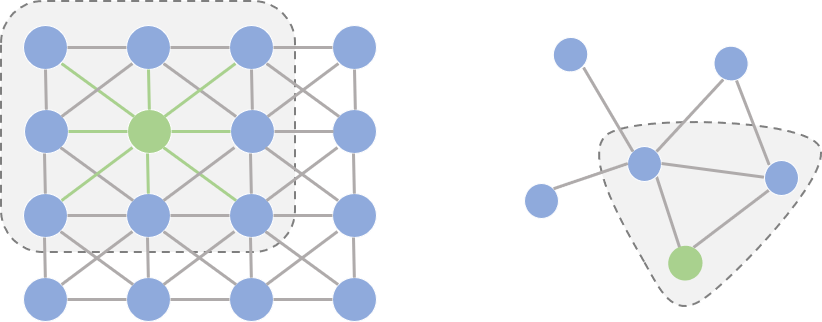• 欧式空间中的卷积操作实际上是用固定大小可学习的卷积核来抽取像素的特征，比如这里就是抽取绿色结点对应像素及其相邻像素点的特征。但是因为图里的邻居结点不固定，所以传统的卷积核不能直接用于抽取图上结点的特征。

• 提出一种方式把非欧空间的图转换成欧式空间。
• 找出一种可处理变长邻居结点的卷积核在图上抽取特征。

## 图卷积框架(Framework)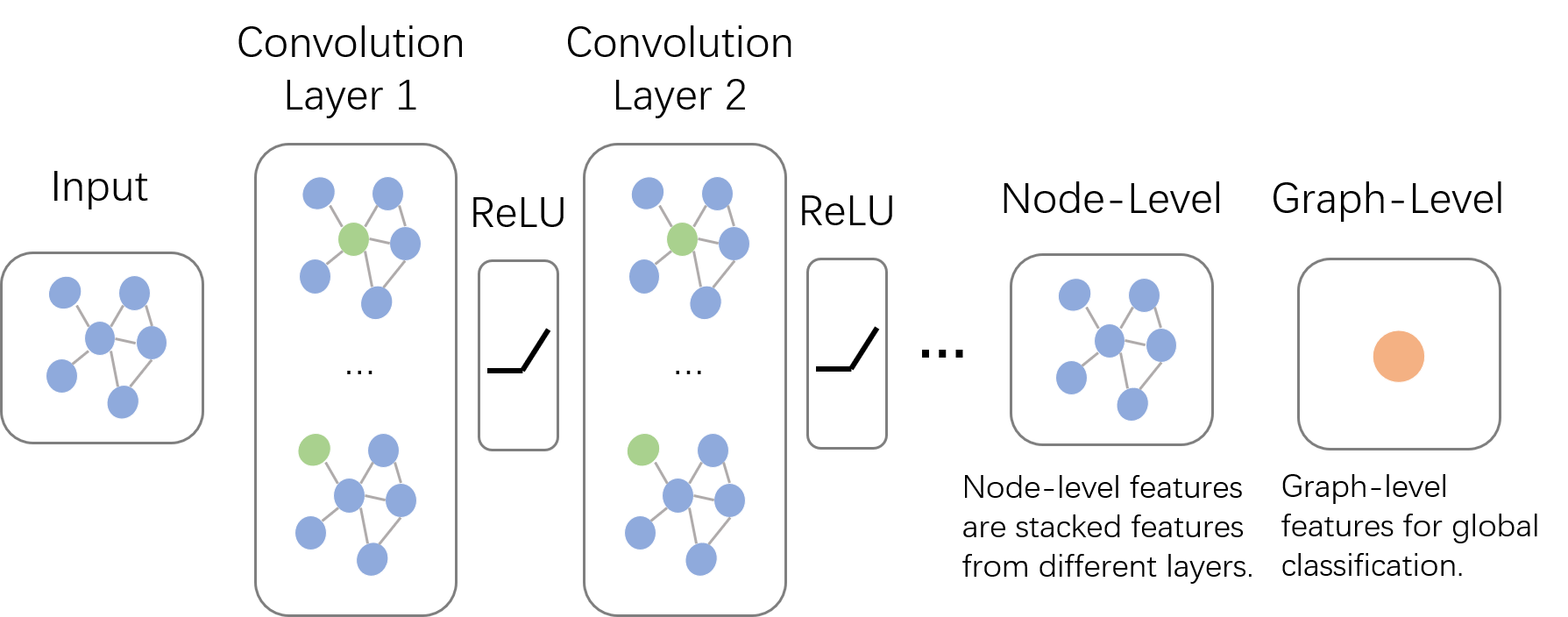## 卷积(Convolution)

### 基础概念

$(f*g)(t)={\int}_{-\infty}^{\infty}f(\tau)g(t-\tau) (连续形式)$

$(f*g)(t)={\sum}_{\tau=-\infty}^{\infty}f(\tau)g(t-\tau) (离散形式)$

### 实例:掷骰子问题

• $$f$$ 向上为1，$$g$$ 向上为3；
• $$f$$ 向上为2，$$g$$ 向上为2；
• $$f$$ 向上为3，$$g$$ 向上为1；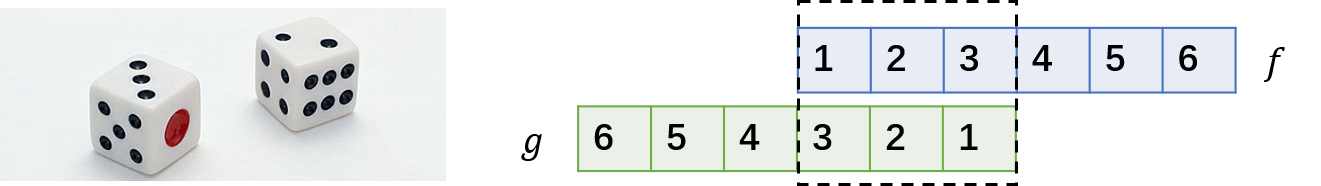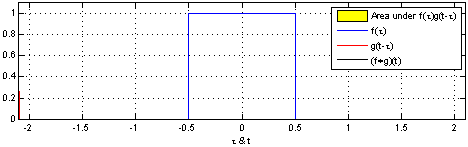## 空域卷积(Spatial Convolution)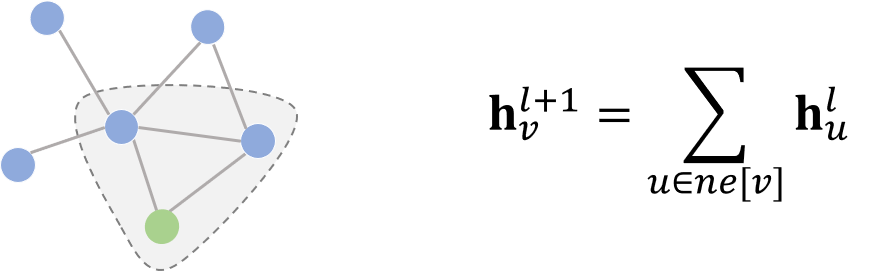### 消息传递网络(Message Passing Neural Network)

$\mathbf{h}_{v}^{l+1}=U_{l+1}(\mathbf{h}_v,\sum_{u{\in}ne[v]}M_{l+1}(\mathbf{h}_v^l,\mathbf{h}_u^l,\mathbf{x}_{vu}))$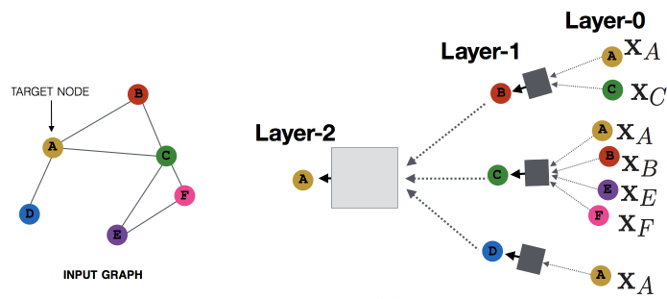### 图采样与聚合(Graph Sample and Aggregate)

MPNN很好地概括了空域卷积的过程，但定义在这个框架下的所有模型都有一个共同的缺陷：卷积操作针对的对象是整张图，也就意味着要将所有结点放入内存/显存中，才能进行卷积操作。但对实际场景中的大规模图而言，整个图上的卷积操作并不现实。GraphSage提出的动机之一就是解决这个问题。从该方法的名字我们也能看出，区别于传统的全图卷积，GraphSage利用采样(Sample)部分结点的方式进行学习。当然，即使不需要整张图同时卷积，GraphSage仍然需要聚合邻居结点的信息，即论文中定义的aggregate的操作。这种操作类似于MPNN中的消息传递过程。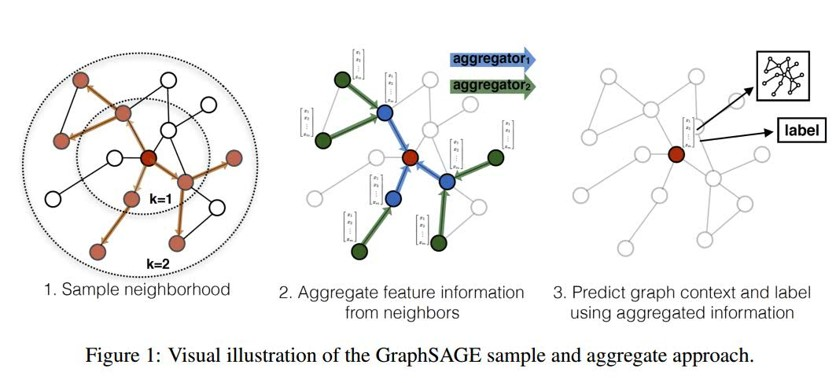1. 在图中随机采样若干个结点，结点数为传统任务中的batch_size。对于每个结点，随机选择固定数目的邻居结点(这里邻居不一定是一阶邻居，也可以是二阶邻居)构成进行卷积操作的图。
2. 将邻居结点的信息通过$$aggregate$$函数聚合起来更新刚才采样的结点。
3. 计算采样结点处的损失。如果是无监督任务，我们希望图上邻居结点的编码相似；如果是监督任务，即可根据具体结点的任务标签计算损失。

$\mathbf{h}_{v}^{l+1}=\sigma(\mathbf{W}^{l+1}\cdot aggregate(\mathbf{h}_v^l,\{\mathbf{h}_u^l\}),{\forall}u{\in}ne[v])$

GraphSage的设计重点就放在了$$aggregate$$函数的设计上。它可以是不带参数的$$max$$, $$mean$$, 也可以是带参数的如$$LSTM$$等神经网络。核心的原则仍然是，它需要可以处理变长的数据。在本系列博客的第三篇笔者会介绍卷积神经网络中针对图任务的ReadOut操作，$$aggregate$$函数的设计与其有异曲同工之妙，此处就不展开叙述了。

### 图结构序列化(PATCHY-SAN)

PATCHY-SAN 通过以下三个步骤来解决这两个问题：

1. 结点选择(Node Squenece Selection)。该过程旨在与通过一些人为定义的规则(如度大的结点分数很高，邻居的度大时分数较高等)为每个结点指定一个在图中的排序。在完成排序后，取出前 $$\omega$$ 个结点作为整个图的代表。
2. 邻居结点构造(Neighborhood graph construction)。在完成结点排序后，以第1步选择的结点为中心，得到它们的邻居(这里的邻居可以是第一阶邻居，也可以是二阶邻居)结点，就构成了 $$\omega$$ 个团。根据第1步得到的结点排序对每个团中的邻居结点进行排序，再取前 $$k$$ 个邻居结点按照顺序排列，即组成 $$\omega$$ 个有序的团。
3. 图规范化(Graph Noermalization)。按照每个团中的结点顺序可将所有团转换成固定长度的序列($$k+1$$)，再将它们按照中心结点的排序从前到后依次拼接，即可得到一个长度为 $${\omega}*(k+1)$$ 的代表整张图的序列。这样，我们就可以直接使用带1D的卷积神经网络对该序列建模，比如图分类(可类比文本序列分类)。值得注意的一点是，在第1步和第2步中，如果取不到 $$\omega$$$$k$$ 个结点时，要使用空结点作填充(padding)。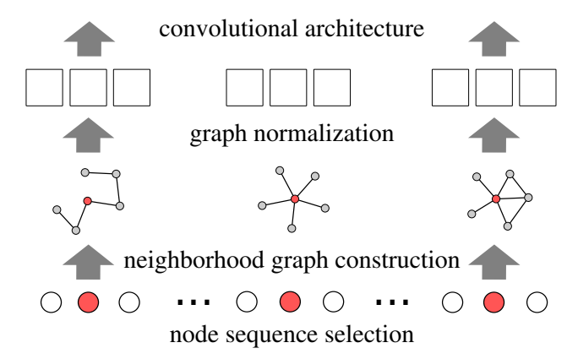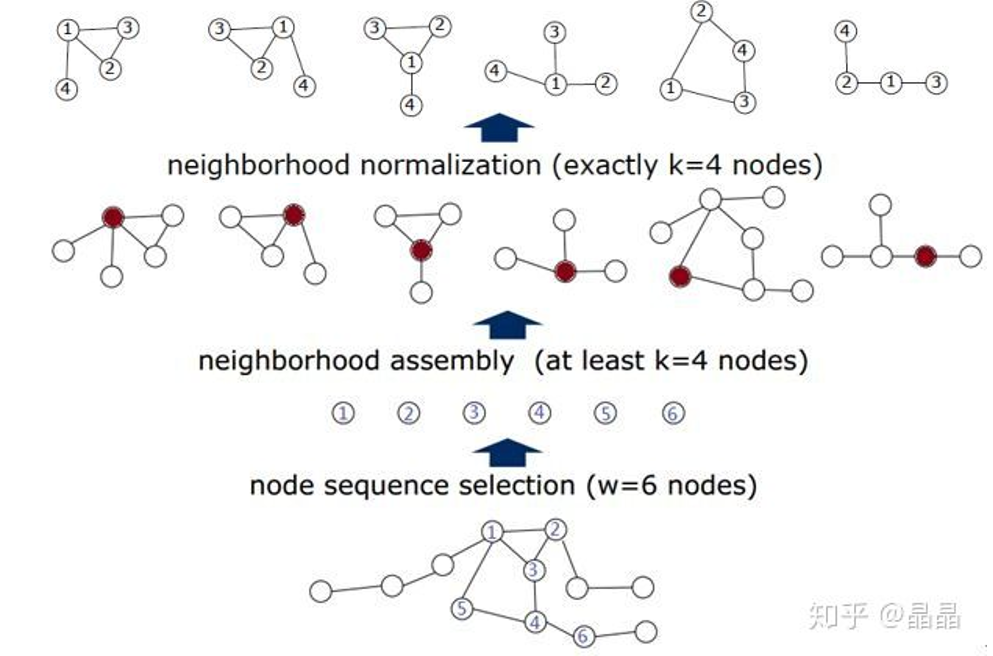## 频域卷积(Spectral Convolution)

### 前置内容

#### 傅里叶变换(Fourier Transform)

$(f*g)(t)=F^{-1}[F[f(t)]{\odot}F[g(t)]]$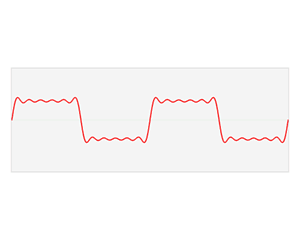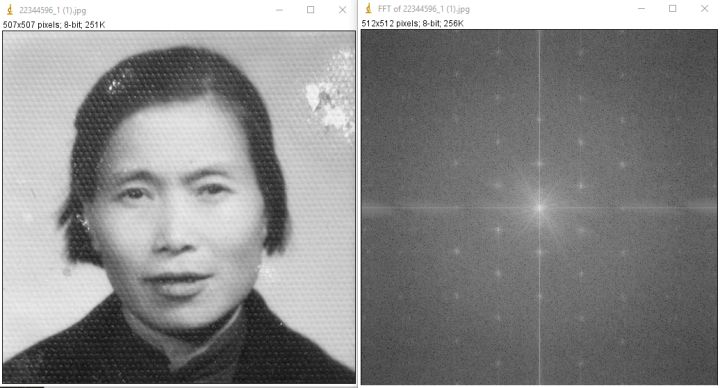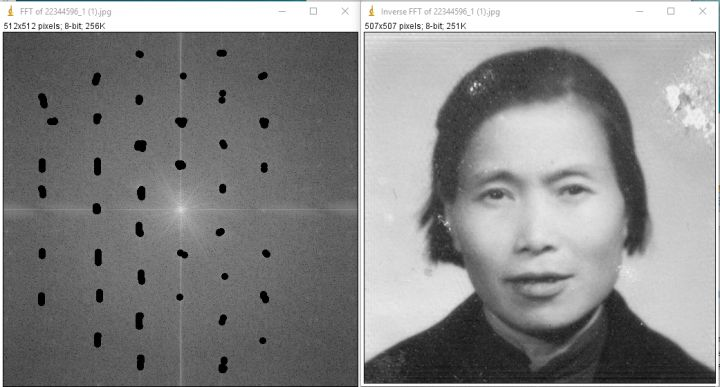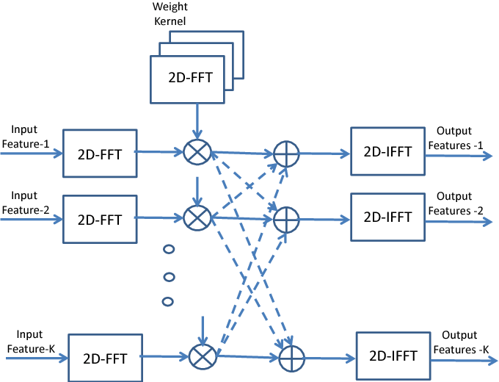$\hat{f}(t)={\int}f(x){\exp}^{-2{\pi}ixt}dx$

$Ax={\lambda}x$

$∆{\exp}^{-2{\pi}ixt}=\frac{{\partial}^2}{{\partial}t^2}{\exp}^{-2{\pi}ixt}={-4{\pi}^2x^2}{\exp}^{-2{\pi}ixt}$

#### 图上的傅里叶变换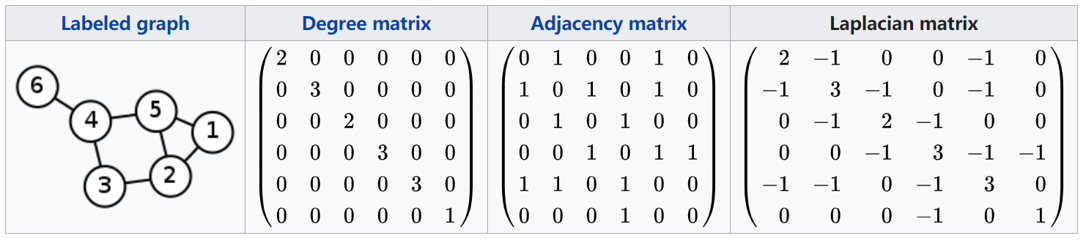$L = U{\Lambda}U^{T}$

$U = (u_1, u_2,{\cdots},u_n)$

${\Lambda}=\left[ \begin{matrix} {\lambda}_1 & ... & 0 \\ ... & ... & ... \\ 0 & ... & {\lambda}_n \end{matrix} \right]$

${\hat{f}}=\left[ \begin{matrix} {\hat{f}}(1) \\ ... \\ {\hat{f}}(N) \end{matrix} \right]=U^Tf$

$(f*g)=F^{-1}[F[f]{\odot}F[g]]$

$(f{*}_{G}g)=U(U^Tf{\odot}U^Tg)=U(U^Tg{\odot}U^Tf)$

$o = (f*_{G}g)_{\theta} = Ug_{\theta}U^Tf$

### 频域卷积网络(Spectral CNN)

$h^{l+1}_{:,j}={\sigma}(U{\sum}_{i=1}^{d_l}{\Theta}^l_{i,j}U^Th^l_{:,i})$

${\Theta}^l_{i,j}=g_{\theta}= \left[ \begin{matrix} {\theta}_1 & ... & 0 \\ ... & ... & ... \\ 0 & ... & {\theta}_N \end{matrix} \right]$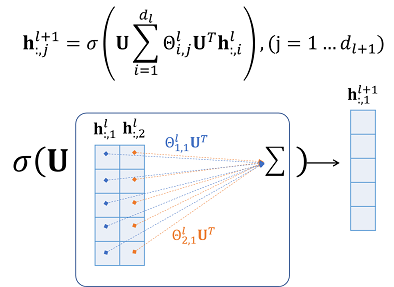### 切比雪夫网络(ChebNet)

$g_{\theta}={\sum}_{k=0}^{K-1}{\theta}_{k}T_{k}(\tilde{\Lambda}), \text{where}\ \tilde{\Lambda}=\displaystyle\frac{2\Lambda}{\lambda_{max}}-I_N$

## 参考文献

. Neural Message Passing for Quantum Chemistry, https://arxiv.org/abs/1704.01212

. Inductive Representation Learning on Large Graphs, https://arxiv.org/abs/1706.02216

. Diffusion-Convolutional Neural Networks, https://arxiv.org/abs/1511.02136

. Learning Convolutional Neural Networks for Graphs, https://arxiv.org/pdf/1605.05273

. Spectral Networks and Locally Connected Networks on Graphs, https://arxiv.org/abs/1312.6203

. Convolutional neural networks on graphs with fast localized spectral filtering, https://papers.nips.cc/paper/6081-convolutional-neural-networks-on-graphs-with-fast-localized-spectral-filtering

. Semi-Supervised Classification with Graph Convolutional Networks, https://arxiv.org/pdf/1609.02907

. 如何通俗易懂地解释卷积, https://www.zhihu.com/question/22298352

. Spectral Networks and Locally Connected Networks on Graphs
, https://arxiv.org/abs/1312.6203

. Convolutional neural networks on graphs with fast localized spectral filtering, https://arxiv.org/abs/1606.09375

posted @ 2019-06-09 22:31  SivilTaram  阅读(91824)  评论(70编辑  收藏  举报